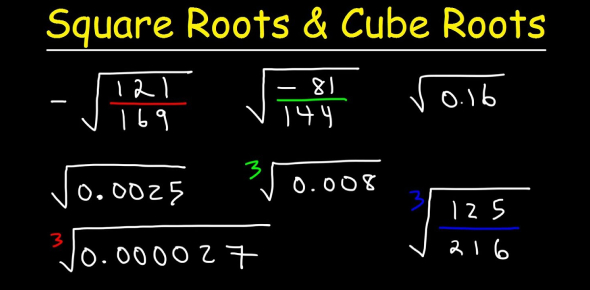# Challenging Quiz On Square And Cube Roots

Approved & Edited by ProProfs Editorial Team
The editorial team at ProProfs Quizzes consists of a select group of subject experts, trivia writers, and quiz masters who have authored over 10,000 quizzes taken by more than 100 million users. This team includes our in-house seasoned quiz moderators and subject matter experts. Our editorial experts, spread across the world, are rigorously trained using our comprehensive guidelines to ensure that you receive the highest quality quizzes.
| By Martin2011
M
Martin2011
Community Contributor
Quizzes Created: 1 | Total Attempts: 10,320
Questions: 6 | Attempts: 10,331SettingsWhen it comes to mathematics, some people have a hard time finding the squares and cube roots for some numbers. For this quiz, you should know how to calculate square and cube roots and find out an approximate square and cube root. Give it a try and all the best!

• 1.

### 1. What is the square root of 144 ?

• A.

11

• B.

60

• C.

12

• D.

13.5

C. 12
Explanation
The square root of a number is a value that, when multiplied by itself, gives the original number. In this case, the square root of 144 is 12 because 12 multiplied by itself equals 144.

Rate this question:

• 2.

### 2. What is the square root of 96 ?

• A.

Between 8 and 9

• B.

Between 9 and 10

• C.

11

• D.

Between 4 and 5

B. Between 9 and 10
Explanation
The square root of 96 is between 9 and 10 because when we calculate the square root of 96, we find that it is approximately 9.8. Since 9.8 falls between 9 and 10, the correct answer is between 9 and 10.

Rate this question:

• 3.

### 3. If the answer is 5 what is the cube root ?

• A.

120

• B.

25

• C.

125

• D.

100

C. 125
Explanation
The cube root of 125 is 5 because when you multiply 5 by itself three times (5 x 5 x 5), you get 125. The cube root is the number that, when multiplied by itself three times, equals the given number.

Rate this question:

• 4.

### 4.the square root of 37 is 6. True or false?

• A.

True

• B.

False

B. False
Explanation
The statement is false because the square root of 37 is not equal to 6. The square root of 37 is actually an irrational number, approximately equal to 6.08. Therefore, the correct answer is false.

Rate this question:

• 5.

### 5.if 6 is the answer what is the square root ?

• A.

12

• B.

36

• C.

27

• D.

18

B. 36
Explanation
The square root of 36 is 6.

Rate this question:

• 6.

### 6.the cube root of 216 is 6. true or false?

• A.

True

• B.

False

A. True
Explanation
The cube root of a number is the value that, when multiplied by itself three times, gives the original number. In this case, if we calculate the cube root of 216, it is indeed 6 because 6 multiplied by itself three times equals 216. Therefore, the statement "the cube root of 216 is 6" is true.

Rate this question:

Related TopicsBack to top